AR theory fundamentals

To understand how an AR can be generated, we first need to understand a number of fundamental concepts in AR theory. These concepts are briefly described below.

Concentration vectors
01
Mixing
02
Convex hulls
03
Rate vectors
04
Fundamental reactor types
05

# Concentration vectors

AR theory is geometric in nature and thus we always seek to represent our system, and the data generated from this system, using vectors.

Consider a beaker containing an aqueous mixture of two components, A and B, where the concentrations of each component are given by $c_{\mathrm{A}}$ and $c_{\mathrm{B}}$. The values of $c_{\mathrm{A}}$ and $c_{\mathrm{B}}$ can be combined into a vector, $\mathbf{C}$, which in AR theory is called the concentration vector:

$$\mathbf{C}=\begin{bmatrix}c_{\mathrm{A}}\\ c_{\mathrm{B}} \end{bmatrix}$$

Any mixture of A and B can be represented compactly as a vector with a unique magnitude and direction. The dimension of the concentration vector is determined by the number of individual species present in the mixture. Thus, for an $n$-component system, the concentration vector concept may be generalised to give:

$$\mathbf{C}=\begin{bmatrix}c_{A}\\ c_{B}\\ \vdots\\ c_{n} \end{bmatrix}$$

Concentration vectors make it easy to represent mixtures containing many components compactly. Furthermore, since $\mathbf{C}$ is a vector, the concentration of the mixture can be visualised as a unique coordinate in $c_{\mathrm{A}}$-$c_{\mathrm{B}}$ space.

## Example 1

A mixture containing 1 mol/L A and 2.5 mol/L B is represented by the concentration vector $\mathbf{C}_{1}=\begin{bmatrix}1.0 & 2.5\end{bmatrix}^{\mathrm{T}}$ mol/L. This vector can be plotted as the point (1.0, 2.5) in $c_{\mathrm{A}}$-$c_{\mathrm{B}}$ space. If a second beaker containing 6.0 mol/L A and 8.0 mol/L B were also available, then this mixture is given by the vector $\mathbf{C}_{2}=\begin{bmatrix}6.0 & 8.0\end{bmatrix}^{\mathrm{T}}$ mol/L, and the coordinate (6.0, 8.0) in $c_{\mathrm{A}}$-$c_{\mathrm{B}}$ space.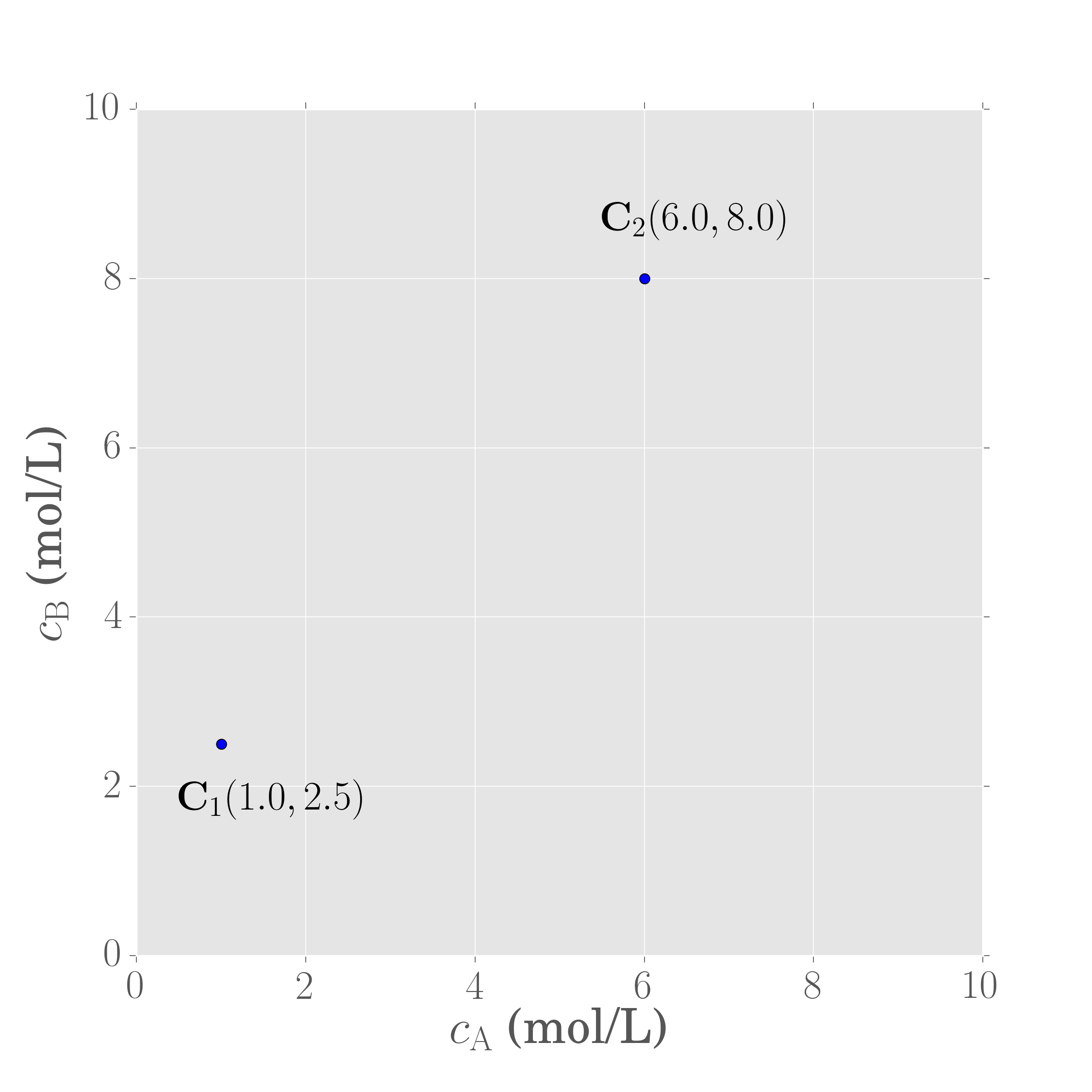Had the mixture contained three components instead of two, we could still apply the idea of concentration vectors. Although, for three components, the system is now three-dimensional, and thus the concentration vectors are represented as coordinates in three-dimensional conentration space.

## Example 2

Consider now two beakers that each hold a mixture containing three components, A, B and C in the following proportions:

• Beaker 1: 1.0 mol/L A; 2.5 mol/L B; 0.5 mol/L C.
• Beaker 2: 6.0 mol/L A; 8.0 mol/L B; 3.1 mol/L C.

The concentration vectors $\mathbf{C}_1$ and $\mathbf{C}_2$ belonging to these two mixtures would then be

$$\mathbf{C}_{1}=\begin{bmatrix}1.0\\ 2.5\\ 0.5 \end{bmatrix}\,\mathrm{mol/L}$$

and

$$\mathbf{C}_{2}=\begin{bmatrix}6.0\\ 8.0\\ 3.1 \end{bmatrix}\,\mathrm{mol/L}$$

$\mathbf{C}_1$ and $\mathbf{C}_2$ are now three-dimensional (because there are three components in the mixture). Plotting the concentration vectors results in two points in three-dimensional concentration space.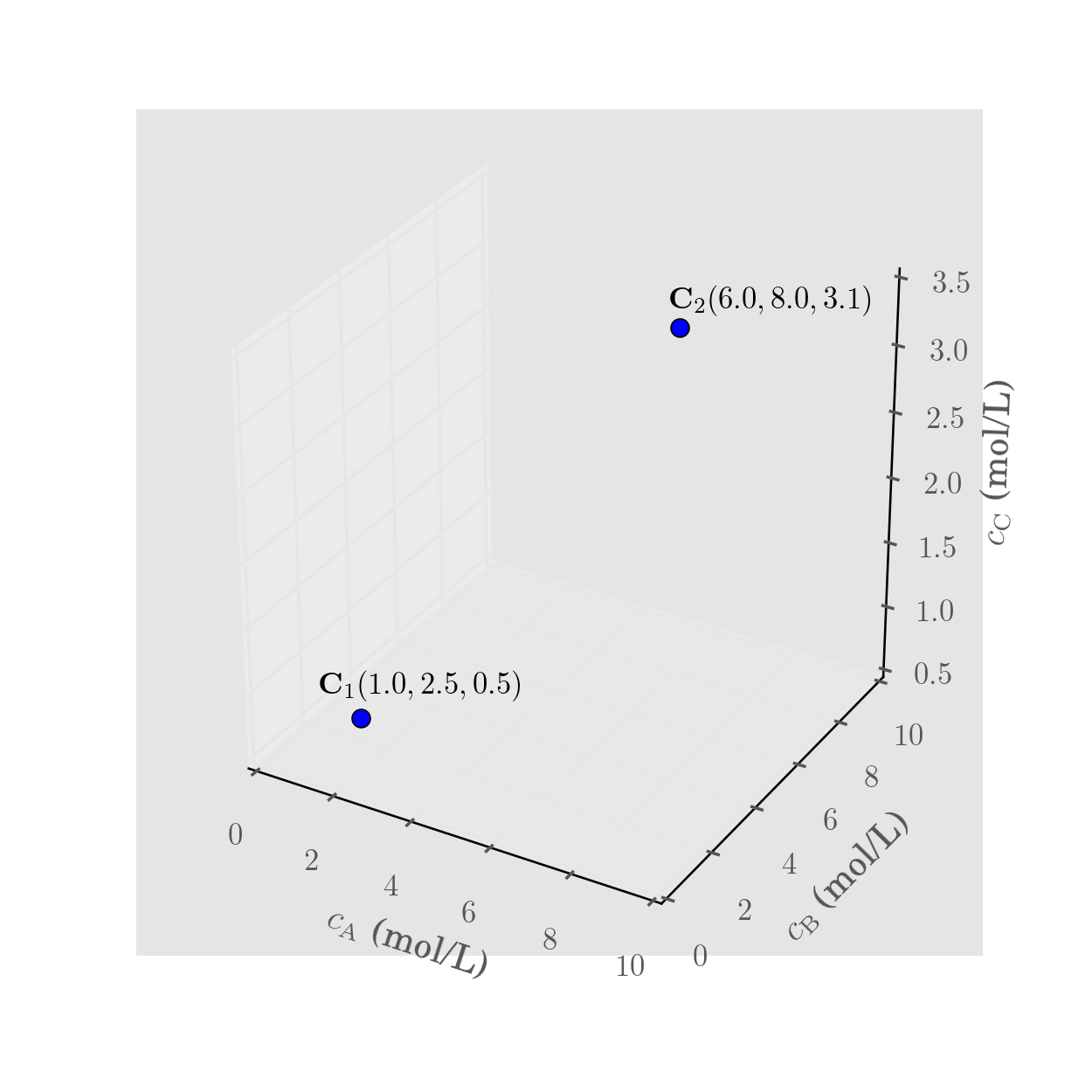Note that the concentration vector concept is consistent for any positive number of components — a 52 component mixture (where $n=52$) would correspond to a unique point in 52 component concentration space, for instance.

# Mixing

Imagine now that beakers 1 and 2 from the previous section are mixed together. Let us assume that beaker 1 has a volume $V_1$ and beaker 2 has a volume $V_2$, and that the density of the mixture is constant (so that volumes can be added together). A mass balance can be performed to find the concentration of the mixture, $\mathbf{C}^*$.

$$\mathbf{C}^* = \frac{V_1\mathbf{C}_1 + V_2\mathbf{C}_2}{V_1 + V_2}$$

If the following substitution is made:

$$\lambda = \frac{V_1}{V_1 + V_2}$$

The expression describing the concentration of the mixture can be simplified to give:

$$\mathbf{C}^* = \lambda\mathbf{C}_1 + \left(1 - \lambda\right)\mathbf{C}_2$$

which shows that $\mathbf{C}^*$ is simply a linear combination of beaker concentrations, $\mathbf{C}_1$ and $\mathbf{C}_2$. Note that $\lambda$ is a scalar quantity, whereas $\mathbf{C}_1$, $\mathbf{C}_2$ and $\mathbf{C}^*$ are vector quantities. Geometrically, mixing any two concentrations together, involves drawing a straight line between the two terminal concentrations because the final mixture concentration must lie on a straight line joining $\mathbf{C}_1$ and $\mathbf{C}_2$, as is shown below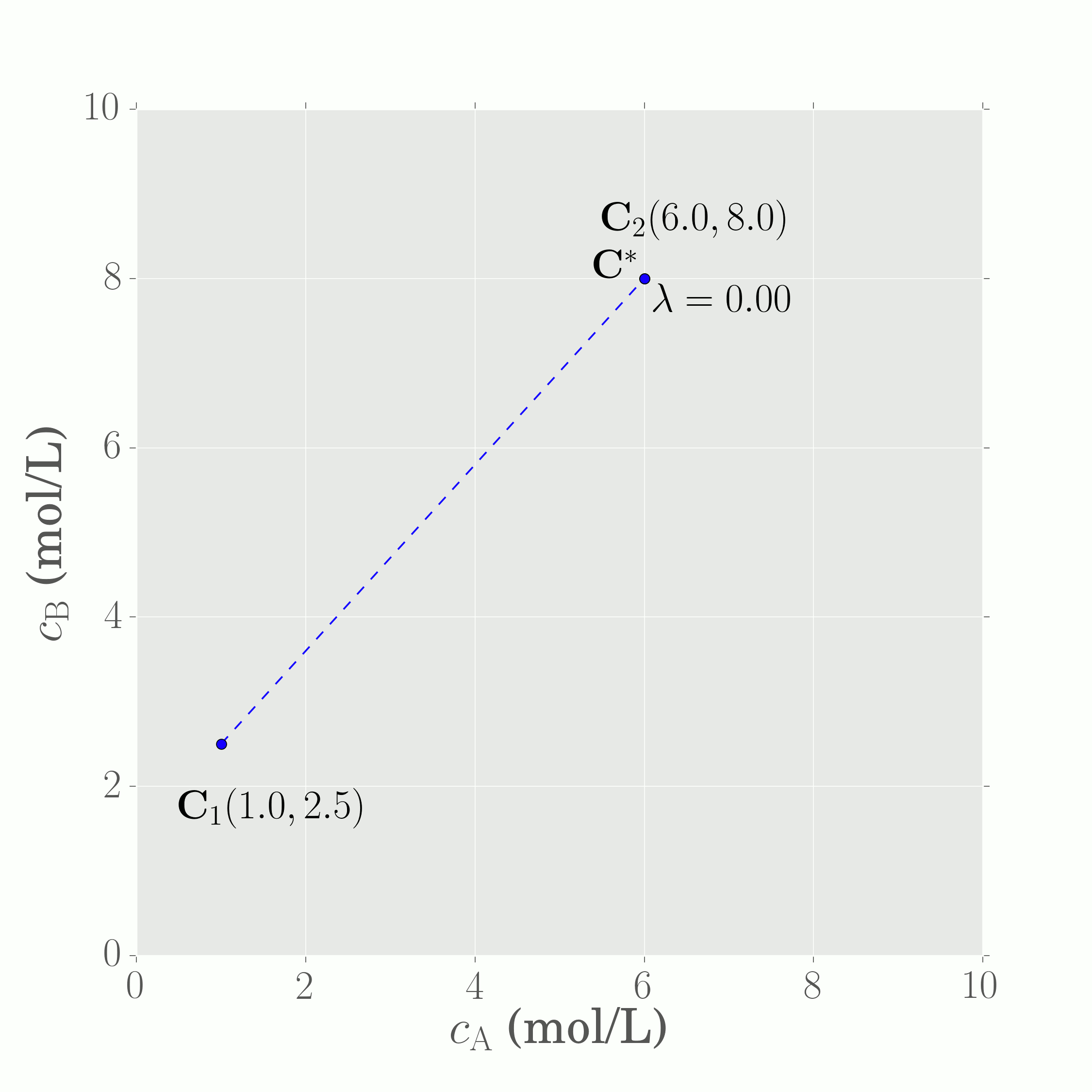When the value of $\lambda = 0$, $\mathbf{C}^{*} = \mathbf{C}_2$ and the mixture concentration is the same as the concentration of beaker 2. Similarly, when $\lambda = 1$, $\mathbf{C}^{*} = \mathbf{C}_1$, indicating that the mixture is composed entirely of the contents of beaker 1. For any value of $\lambda$ between zero and one, the mixture concentration is determined by a linear combination of both beaker concentrations, with the exact mixture concentration dictated by the specific value of $\lambda$.

# Convex hulls

Suppose two extra mixtures of A and B are available, so that there are now four beakers and thus four concentration vectors available for mixing: $\mathbf{C}_1$, $\mathbf{C}_2$, $\mathbf{C}_3$ and $\mathbf{C}_4$. All four concentration vectors may be plotted as coordinates in $c_{\mathrm{A}}$-$c_{\mathrm{B}}$ space, which is shown below.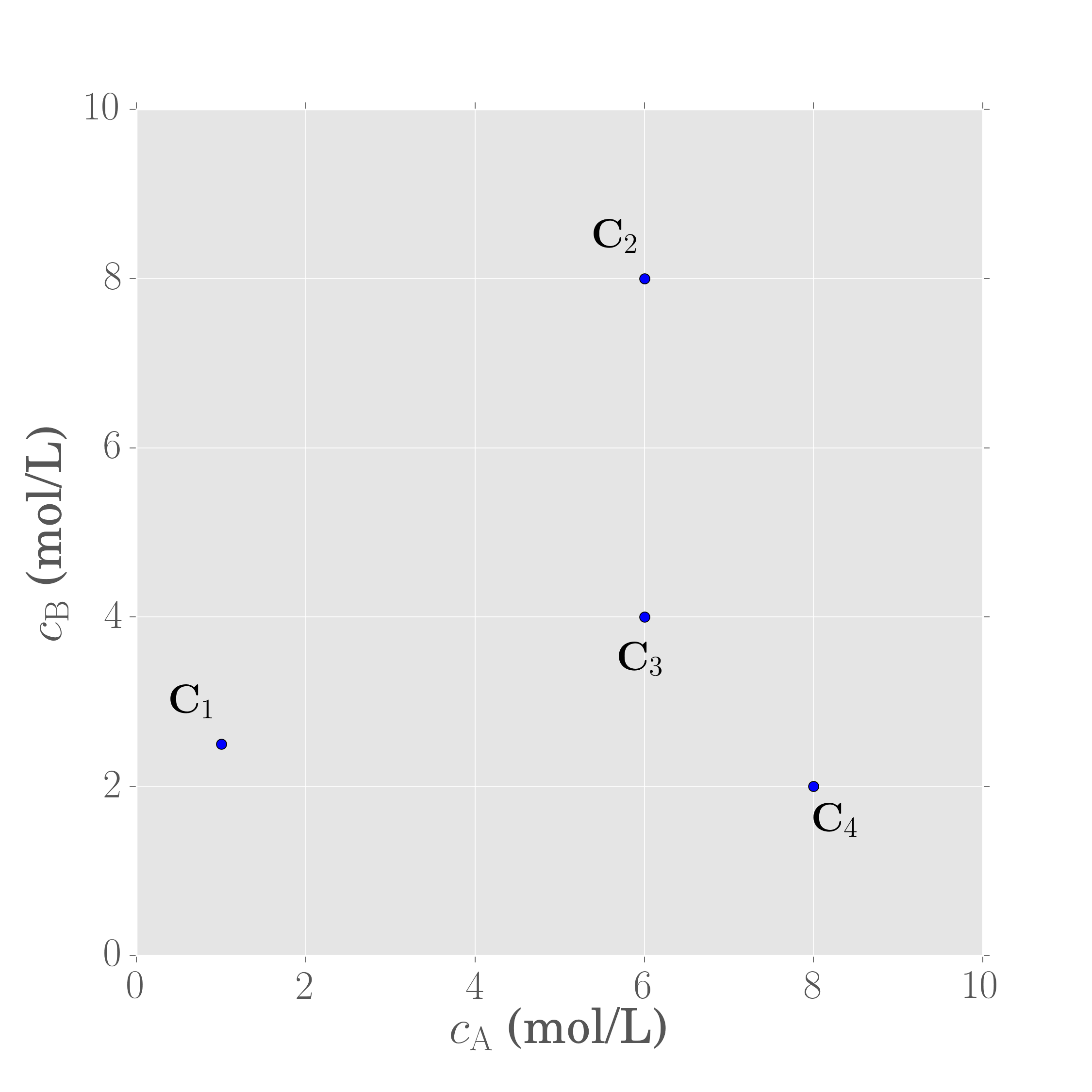What kinds of mixture concentrations are achievable from the four beakers? Since it is now understood that mixing is a (geometrically) linear process, which is equivalent to drawing straight line segments between the two concentrations that are being mixed, one method of determining achievable concentrations would be to first construct mixing lines between the initial set of four concentrations, and then to draw additional mixing lines that extend from the first set of mixture concentrations. By example, one might mix beaker 1 with beaker 3, mix beaker 2 with beaker 4, mix beaker 2 with the mixture of beaker 1 and 3, and so on.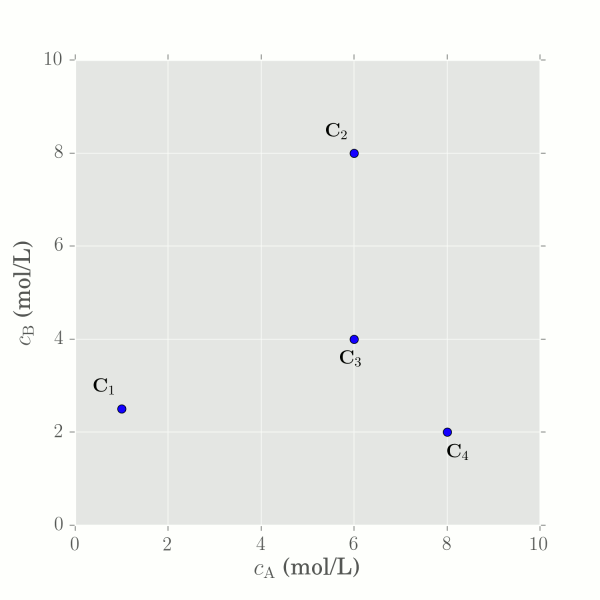If this process is performed enough times with different beaker combinations and mixing ratios, we begin to see that the initial four beaker concentrations generate mixtures that fill out a region in concentration space. This region is well-defined and finite (because it does not extend infinitely in all directions). Yet, there are still an infinite number of mixtures that can be formed from these four concentrations even though the region is finite. Notice that all mixture concentrations formed inside the region result from combinations of the initial perimeter concentrations. In the present example, $\mathbf{C}_1$, $\mathbf{C}_2$ and $\mathbf{C}_4$ are the perimeter concentrations that can generate all concentrations in the region.

The three perimeter concentrations are significant because these can be used to generate all mixture concentrations in the region. They constitute what is called the convex hull of the set of points.

## Convex hull definition

The convex hull of a set of points, $\mathbb{X}$, is the smallest convex set that contains (encompasses) $\mathbb{X}$. In the current example, $\mathbb{X}$ is the set of vectors $\left\{\mathbf{C}_{1},\mathbf{C}_{2},\mathbf{C}_{3},\mathbf{C}_{4}\right\}$, but the convex hull of $\mathbb{X}$ only contains $\mathbf{C}_1$, $\mathbf{C}_2$ and $\mathbf{C}_4$, as $\mathbf{C}_3$ is enclosed in the region defined by the other three points. Geometrically, $\mathbf{C}_3$ is an interior point, whereas $\mathbf{C}_1$, $\mathbf{C}_2$ and $\mathbf{C}_4$ are extreme points of the set.

## Useful properties of convex hulls

The convex hull is a common mathematical concept, and convex hulls are a fundamental concept of AR theory. Convex hulls allow us to think about how a finite set of points can be transformed into a region containing infinitely many mixtures. Mixing, and by extension convex hulls, allow us to generate new concentrations by connecting previously achievable concentrations together, and filling in space. Two useful properties of mixing, and by extension convex hulls, are:

### 1) Mixing creates convex regions from concave regions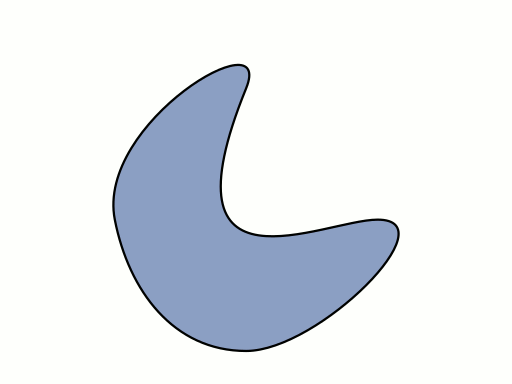Since we can always connect two achievable concentrations together with straight lines representing mixing, the resulting region from mixing must always be convex -- it cannot contain concavities because any concavities can be filled in with mixing lines.

### 2) Mixing fills in voids and connects many separate (achievable) regions together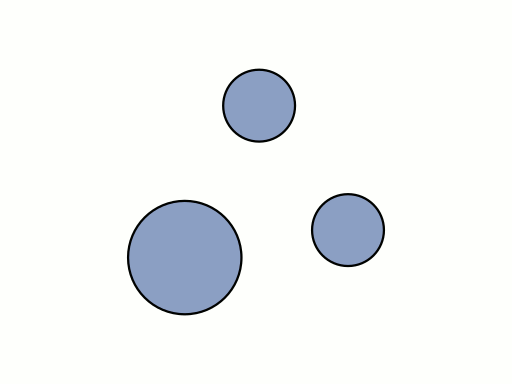Given many individual, convex, regions in concentration space, we can mix between the different regions and create a single convex region that encloses all the smaller regions.

# Rate vectors

So far, we have looked at concentration vectors and mixing. The process of mixing is also related to a special vector that we call the mixing vector. We can extend the idea of vectors to include reaction as well. To understand this statement, let us consider the following set of reactions:

### Mixing vectors

Recall the (vector) equation describing the concentration of a mixture, $\mathbf{C}^*$, from two other mixtures, $\mathbf{C}_1$ and $\mathbf{C}_2$, is given by:

$$\mathbf{C^{*}}=\lambda\mathbf{C_{1}}+\left(1-\lambda\right)\mathbf{C_{2}}$$

Factorising this equation for $\lambda$ gives:

$$\mathbf{C}^* = \mathbf{C}_2 + \lambda\left(\mathbf{C}_1 - \mathbf{C}_2\right)$$

This form of the same equation shows that $\mathbf{C}^*$ is expressed in terms of two vectors: an initial starting concentration $\mathbf{C}_2$, and a mixing vector, $(\mathbf{C}_1 - \mathbf{C}_2)$. We can graphically show this result below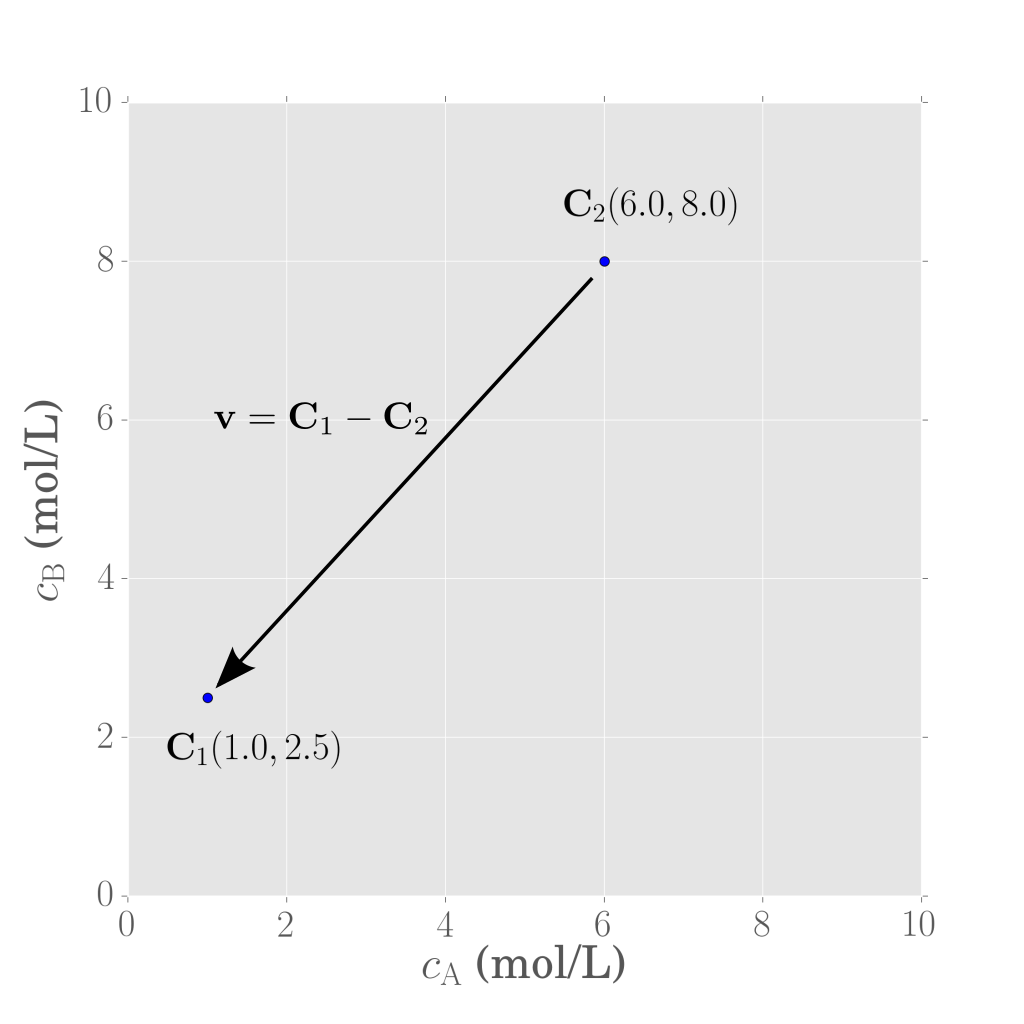The vector difference $\left(\mathbf{C}_1 - \mathbf{C}_2\right)$ is often called the mixing vector, $\mathbf{v}$:

$$\mathbf{v} = \mathbf{C}_1 - \mathbf{C}_2$$

or

$$\mathbf{C^{*}}=\mathbf{C_{2}}+\lambda\mathbf{v}$$

$$\mathrm{A} \rightarrow \mathrm{B}\rightarrow\mathrm{C} \\ 2\mathrm{A} \rightarrow \mathrm{D}$$

with reaction rate epxressions for components A and B given by

\begin{align} r_{\mathrm{A}} &= -k_{1}c_{\mathrm{A}}-2k_{3}c_{\mathrm{A}}^{2} \\ r_{\mathrm{B}} &= k_{1}c_{\mathrm{A}}-k_{2}c_{\mathrm{B}} \end{align}

In general, the rate expression for component $i$ in the reaction is a function of all components in the system. Hence, for each component we can write the component rate expression as a function of the concentration vector $\mathbf{C}$.

$$r_{i}=r_{i}\left(c_{\mathrm{A}},c_{\mathrm{B}},\cdots,c_{n}\right)=r_{i}\left(\mathbf{C}\right)$$

These component rate expressions can then be arranged into another vector, which in AR theory is called the rate vector

$$\mathbf{r}\left(\mathbf{C}\right)=\begin{bmatrix} r_{\mathrm{A}}\left(\mathbf{C}\right)\\ r_{\mathrm{B}}\left(\mathbf{C}\right)\\ \vdots\\ r_{n}\left(\mathbf{C}\right)\end{bmatrix}$$

the vector $\mathbf{r}\left(\mathbf{C}\right)$ is special because it is a vector valued function of the concentration vector — it is a function of a vector ($\mathbf{C}$) that computes a vector as its result.

## Rate fields

For each point $\mathbf{C}$ in concentration space, we can assign a unique rate vector $\mathbf{r}\left(\mathbf{C}\right)$ at $\mathbf{C}$. If all of these rate vectors are plotted over each other in concentration space, we can visualise the rate field corresponding to the reaction kinetics studied.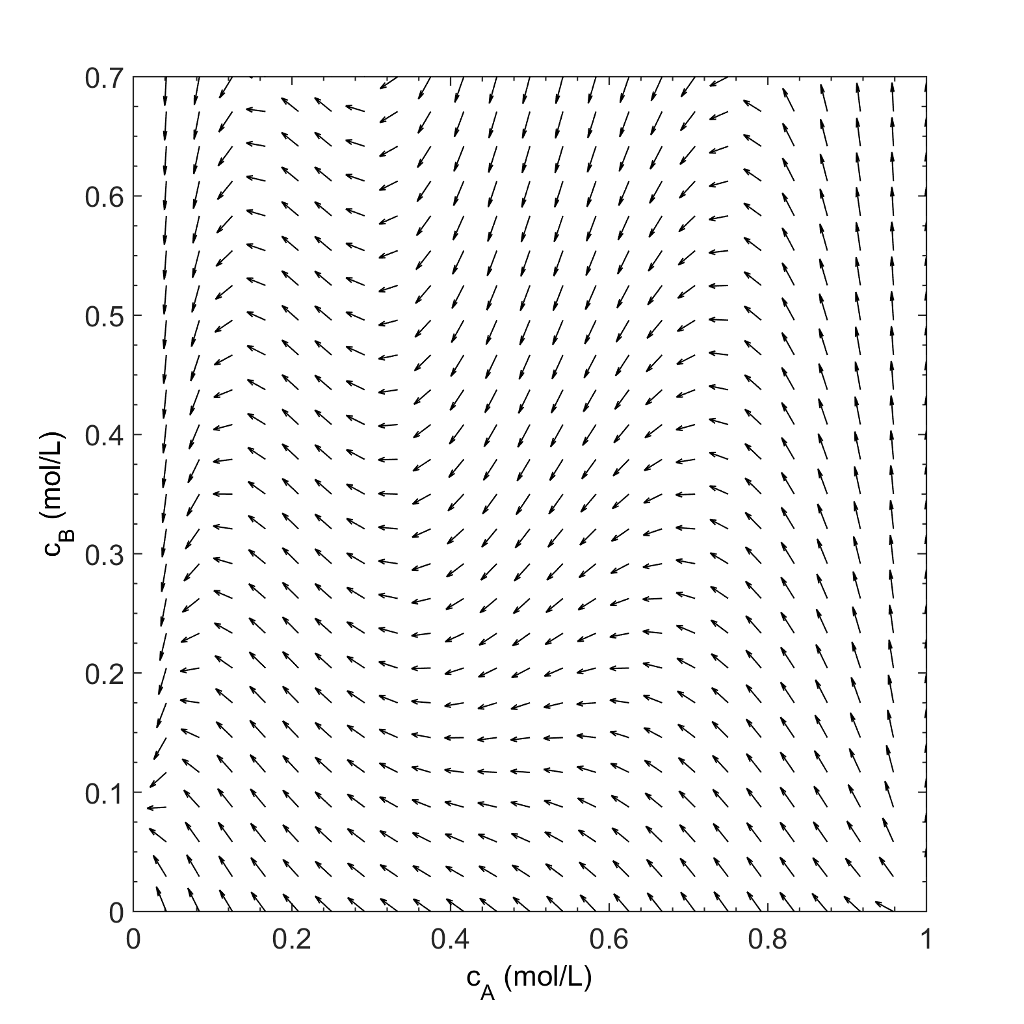Different kinetics will produce different rate fields in concentration space. And different reactor types will interact with the rate field in their own distinct way.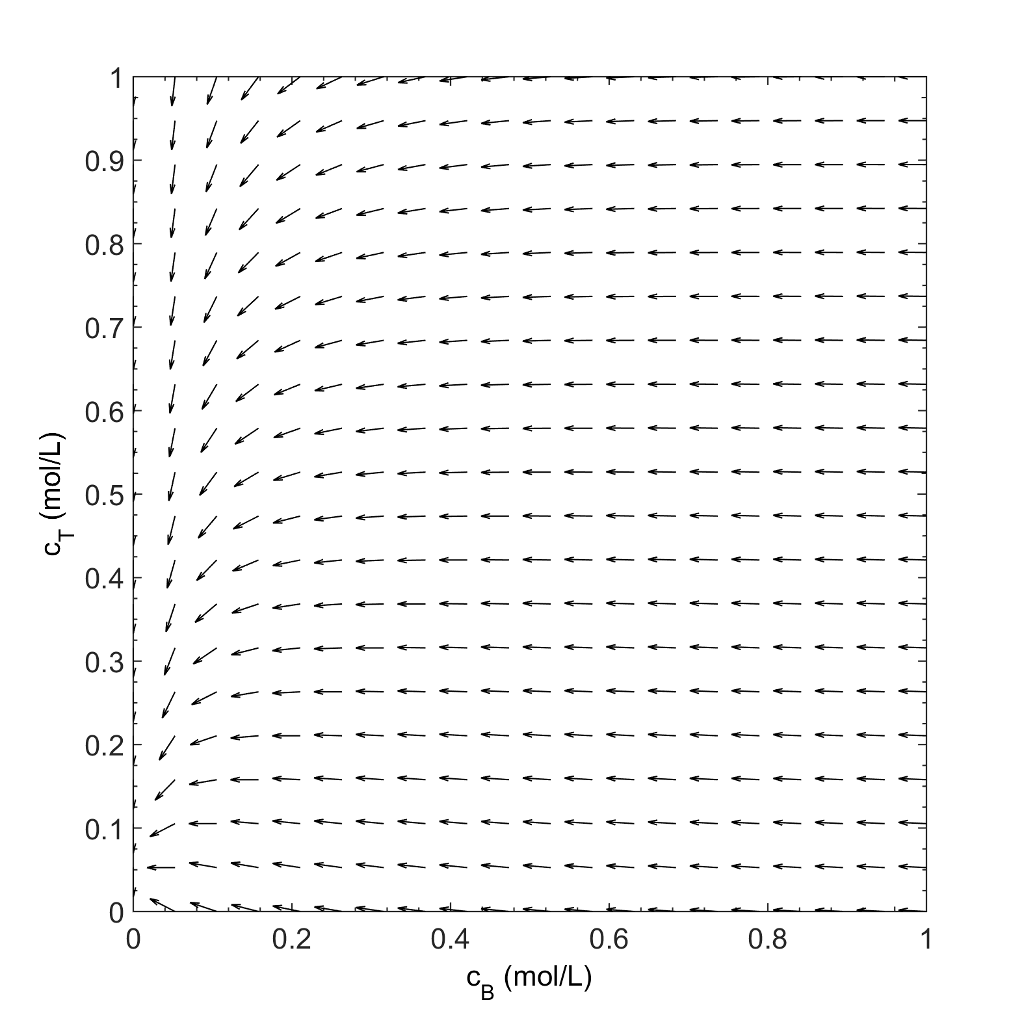# Fundamental reactor types

Although there are many different types of reactors in existence, which could all be used in a reactor network, AR theory only requires the use of three fundamental reactor types. These reactors are characterised by the amount of mixing employed. Use of these three reactors alone allows us to generate attainable regions for all reaction types and any number of components.

## Plug Flow Reactor (PFR)

The plug flow reactor is a reactor type that is commonly used in industry, and is also a vital part of AR theory. We can describe the evolution of component concentrations inside a PFR as a function of residence time, $\tau$, by

$$\frac{\mathrm{d}c_{i}}{\mathrm{d}\tau}=r_{i}\left(\mathbf{C}\right)$$

If there are n-components participating in the reaction, then n PFR equations may be written out corresponding to each component:

\begin{align} \frac{\mathrm{d}c_{A}}{\mathrm{d}\tau} &= r_{A}\left(\mathbf{C}\right) \\ \frac{\mathrm{d}c_{B}}{\mathrm{d}\tau} &= r_{B}\left(\mathbf{C}\right) \\ &\vdots \\ \frac{\mathrm{d}c_{n}}{\mathrm{d}\tau} &= r_{n}\left(\mathbf{C}\right) \end{align}

which can be combined into a vector equation as

$$\begin{bmatrix} \frac{\mathrm{d}c_{\mathrm{A}}}{\mathrm{d}\tau}\\ \frac{\mathrm{d}c_{\mathrm{B}}}{\mathrm{d}\tau}\\ \vdots\\ \frac{\mathrm{d}c_{n}}{\mathrm{d}\tau} \end{bmatrix}=\begin{bmatrix}r_{\mathrm{A}}\left(\mathbf{C}\right)\\ r_{\mathrm{B}}\left(\mathbf{C}\right)\\ \vdots\\ r_{n}\left(\mathbf{C}\right) \end{bmatrix}$$

and expressed compactly as a vector equation:

$$\frac{\mathrm{d}\mathbf{C}}{\mathrm{d}\tau} = \mathbf{r}\left(\mathbf{C}\right)$$

The above equation represents a system of ordinary differential equations (ODEs). Solution of this system requires an initial condition (which for a PFR is the feed concentration to the reactor) as well as an integration time (which for the PFR is the PFR residence time). Solving the system of ODEs results in a PFR solution trajectory. Graphically, PFR trajectories are lines in concentration space that track the concentrations achieved in the PFR along the length of the reactor.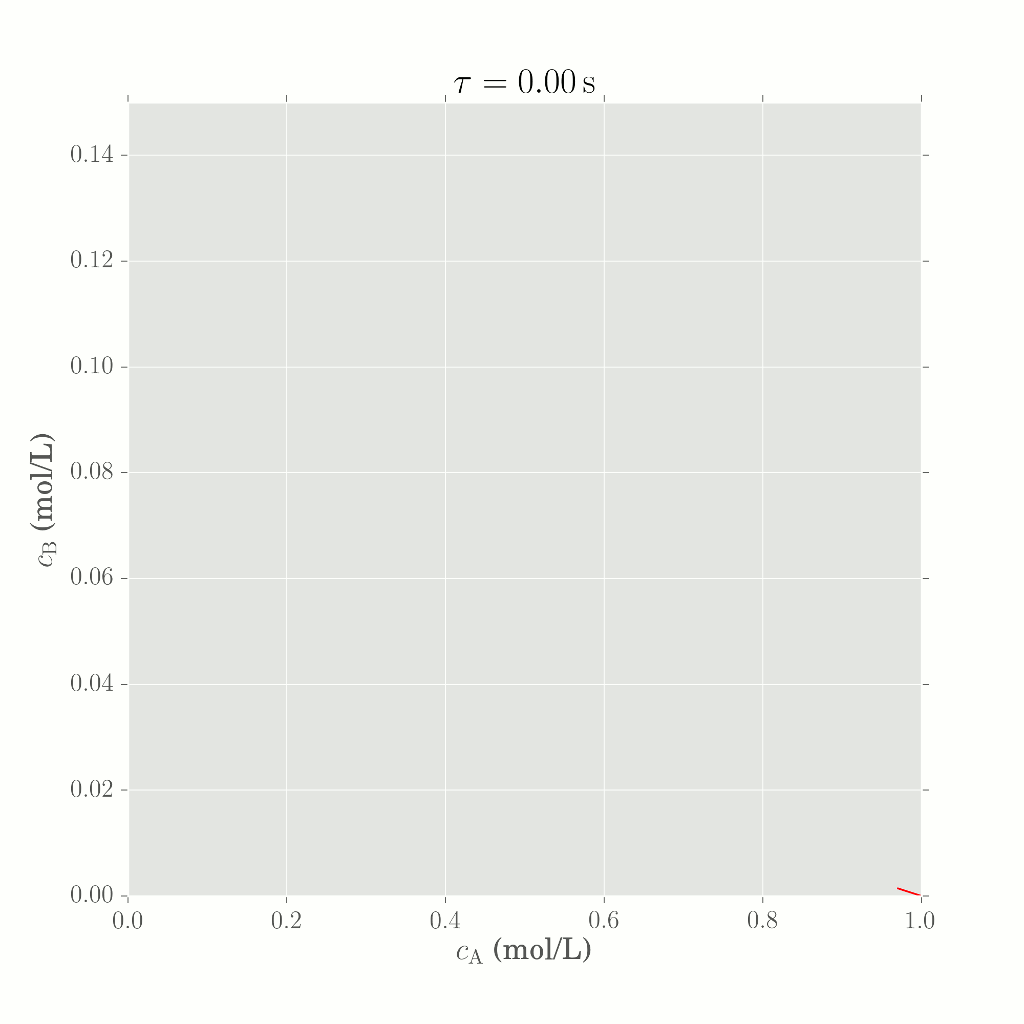### Geometric significance

The term $\mathrm{d}\mathbf{C}/\mathrm{d}\tau$ describes the gradient of the PFR trajectory with respect to PFR residence time evaluated at $\mathbf{C}$, whereas $\mathbf{r}\left(\mathbf{C}\right)$ is the rate vector also evaluated at $\mathbf{C}$. This means that the rate vector evaluated at any point along a PFR solution trajectory is tangent to the trajectory.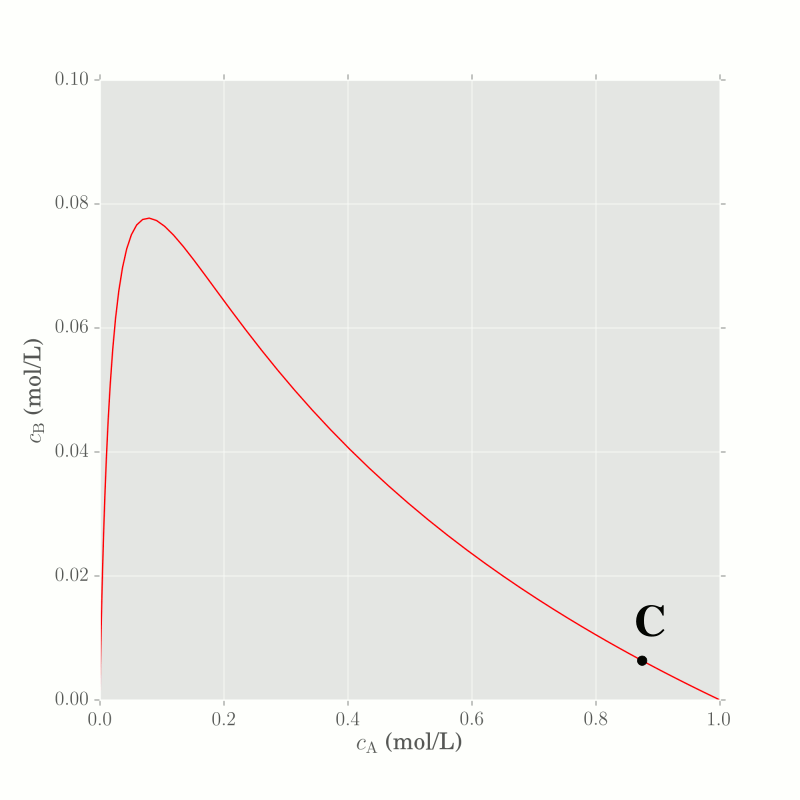## Continuous-flow Stirred Tank Reactor (CSTR)

A component mass balance over a CSTR gives

$$c_{i}=c_{i\mathrm{f}}+\tau r_{i}\left(\mathbf{C}\right)$$

For n components, we can group the system of CSTR equations

$$\begin{bmatrix}c_{A}\\ c_{B}\\ \vdots\\ c_{n} \end{bmatrix}=\begin{bmatrix}c_{\mathrm{Af}}\\ c_{\mathrm{Bf}}\\ \vdots\\ c_{n\mathrm{f}} \end{bmatrix}+\tau\begin{bmatrix}r_{A}\left(\mathbf{C}\right)\\ r_{B}\left(\mathbf{C}\right)\\ \vdots\\ r_{n}\left(\mathbf{C}\right) \end{bmatrix}$$

which can be written in vector form as:

$$\mathbf{C}=\mathbf{C}_{\mathrm{f}}+\tau\mathbf{r}\left(\mathbf{C}\right)$$

Finding the CSTR effluent concentration $\mathbf{C}$ requires the solution of a system of nonlinear equations.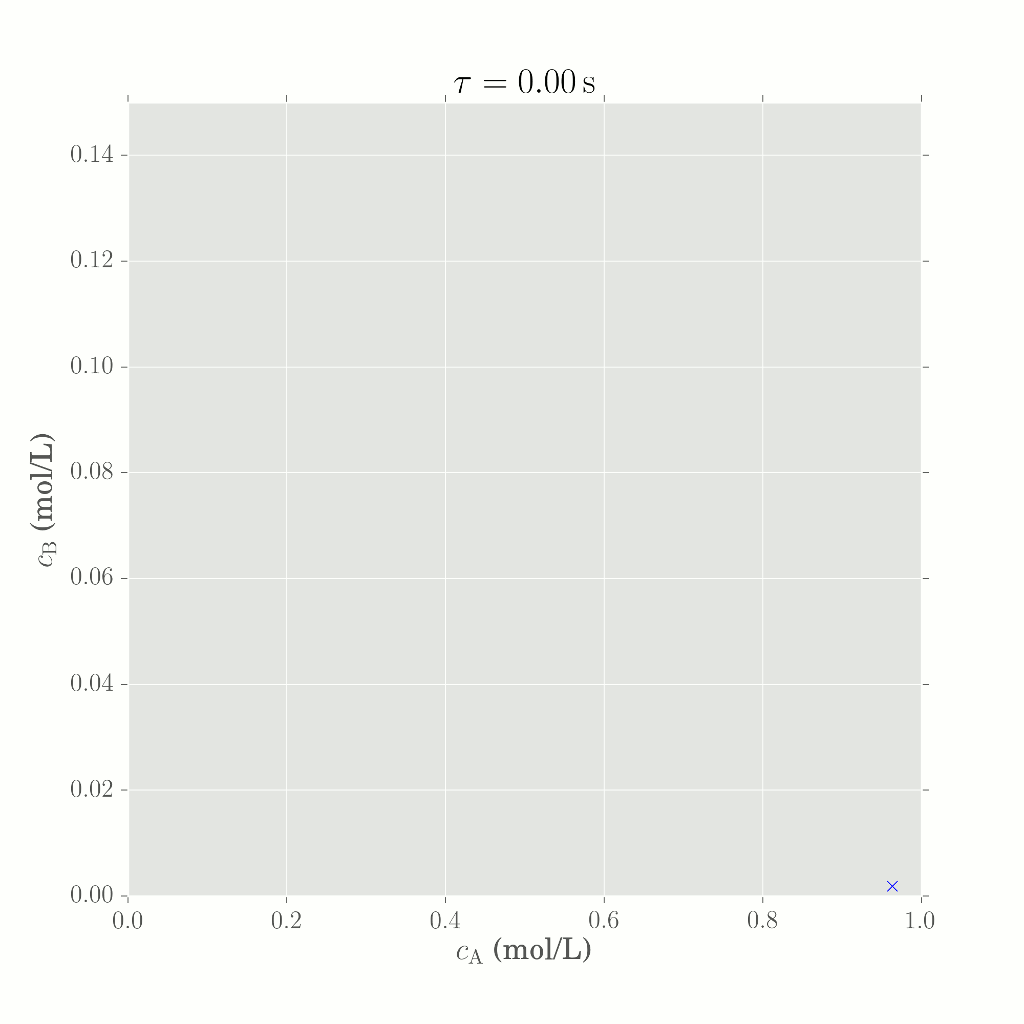### Geometric significance

If the CSTR equation is rearranged

$$\left(\mathbf{C} - \mathbf{C}_{\mathrm{f}}\right) = \tau\mathbf{r}\left(\mathbf{C}\right)$$

Then we can see that the mixing vector $\left(\mathbf{C} - \mathbf{C}_{\mathrm{f}}\right)$ is a scalar multiple of the vector $\mathbf{r}\left(\mathbf{C}\right)$. From a geometric perspective, this result indicates that the rate vector evaluated at a CSTR effluent concentration $\mathbf{C}$, operated with a CSTR feed concentration of $\mathbf{C}_{\mathrm{f}}$, is colinear to the CSTR mixing vector $\left(\mathbf{C} - \mathbf{C}_{\mathrm{f}}\right)$.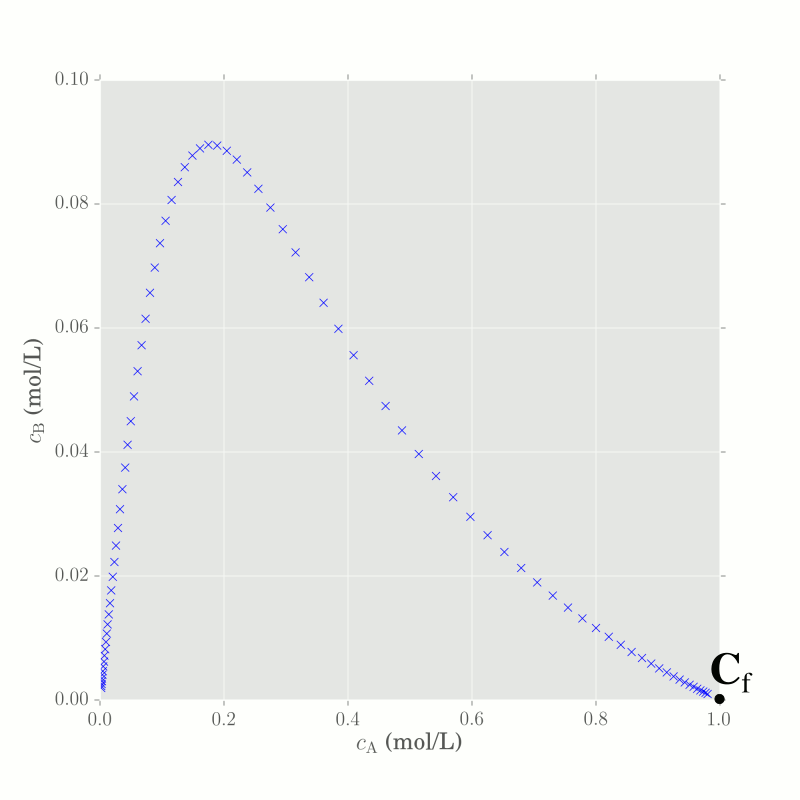## Differential Side-stream Reactor (DSR)

The differential side-stream reactor combines a conventional PFR with additional mixing of fresh material along the length of the reactor. Physically, the DSR is constructed as a PFR with inlet ports along the length of the reactor. This method of operation allows us to control the concentration and thus the rate of reaction inside the DSR, by manipulating the amount and concentration of the side-stream. Due to this type of operation, the volumetric flow rate, Q, inside a DSR increases as we move down the length of the reactor.

The equation for a DSR, in vector form, is given by:

$$\frac{\mathrm{d}\mathbf{C}}{\mathrm{d}\tau} = \mathbf{r}\left(\mathbf{C}\right) + \alpha\left(\mathbf{C}^0 - \mathbf{C}\right)$$

where $\mathbf{C}^0$ is the concentration of the side-stream feeding the DSR and $\alpha$ is a positive scalar that controls the amount of side-stream material that is fed to the DSR. $\alpha$ is often expressed as a function of $\mathbf{C}$ that changes value with $\tau$.

The DSR equation is similar to a PFR in that both equations describe a system of ordinary differential equations that must often be solved via numerical integration. The initial condition used for integration in a DSR is the DSR feed concentration. Solution results in a DSR solution trajectory.

Notice that if $\alpha = 0$ (no side-stream) over the entire DSR integration range, then the DSR equation reduces to the PFR equation.

$$\frac{\mathrm{d}\mathbf{C}} {\mathrm{d}\tau}=\mathbf{r}\left(\mathbf{C}\right)+\mathbf{0}$$

For any positive value of $\alpha$, the direction of movement in concentration space is found by a linear combination of the rate vector $\mathbf{r}\left(\mathbf{C}\right)$ and the DSR mixing vector $(\mathbf{C}^0 - \mathbf{C})$. Thus we can tailor a special trajectory through concentration space by manipulating the value of $\alpha$ along the length of a DSR. In AR theory, DSRs are used to traverse along the AR boundary.